# Nonequilibrium Thermodynamics

## nonequilibrium thermodynamics

[¦nän‚ē·kwə′lib·rē·əm ‚thər·mō·dī′nam·iks]
(thermodynamics)
A quantitative treatment of irreversible processes and of rates at which they occur. Also known as irreversible thermodynamics.

## Thermodynamics, Nonequilibrium

(or irreversible thermodynamics), a general theory of the macroscopic description of nonequilibrium, or irreversible, processes.

Classical thermodynamics studies reversible thermodynamic processes. For nonequilibrium processes it establishes only inequalities that indicate the possible direction of the processes. The main task of nonequilibrium thermodynamics is the quantitative study of nonequilibrium processes, in particular, the determination of their rates in relation to external conditions. Non-equilibrium thermodynamics regards systems in which nonequilibrium processes occur as continuous media and considers the state parameters of such systems to be field variables, that is, continuous functions of the spatial coordinates and time.

In order to carry out the macroscopic description of a nonequilibrium process, the system is represented as consisting of volume elements that are big enough to contain a very large number of molecules. The thermodynamic state of each individual volume element is characterized by temperature, pressure, and other parameters that are used in equilibrium thermodynamics but that here are dependent on the coordinates and time. The quantitative description of nonequilibrium processes in this approach consists in constructing balance equations for the volume elements on the basis of the laws of conservation of mass, momentum, and energy and in constructing the entropy balance equation and phenomenological equations of the processes in question.

The first and second laws of thermodynamics can be formulated for nonequilibrium processes by using the methods of non-equilibrium thermodynamics. In addition, these methods make it possible to obtain from general principles, without considering the details of the mechanism of molecular interactions, a complete system of transport equations—that is, equations of hydrodynamics, heat conduction, and diffusion—for simple and complex systems (for example, systems with chemical reactions between the components and systems in which electromagnetic forces are taken into account).

Law of conservation of mass. For a multicomponent system the rate of change of the mass of the Ath component in a volume element is equal to the mass flux ρkVk into the volume, where ρk is the density and vk the velocity of the component. The change in density for an infinitesimal element of volume is the negative divergence of the mass flux. Consequently, the balance equation for the mass of the kth component has the form ∂ρk/∂t = – div ρkVk. For the overall density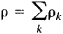the law of conservation has the analogous form ∂ρ/∂t = – div ρv, where v is the medium’s velocity, which is dependent on the coordinates and time. For the concentration of some component ck = ρk/ρ, the law of conservation of mass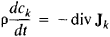permits determination of the diffusion flux Jk = ρk (VkV);here,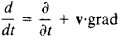is the total derivative with respect to time.

Law of conservation of momentum. A change in the momentum of a volume element can result from forces that are due to the gradient of internal stresses in the medium Pαβ and from external forces Fk. By applying the law of conservation of momentum to volume elements, the fundamental Navier-Stokes equations of hydrodynamics can be obtained:Here, the Vα are the Cartesian components of the velocity v, and Pβα is the stress tensor.

Law of conservation of energy. The law of conservation of energy for volume elements is the first law of thermodynamics in nonequilibrium thermodynamics. Here it is taken into consideration that the total specific energy consists of the specific kinetic and specific potential energies in the force field Fk and the specific internal energy u, which is the energy of thermal motion of the molecules and the average energy of molecular interactions. A balance equation analogous to (1) is obtained for u. It follows from this equation that the rate of change of momentum density per particle ∂ρu/∂t is determined by the divergence of the flux densities of internal energy ρuv and heat Jq and by the work done by the internal stresses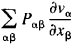and by the applied forces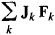Entropy balance equation. In nonequilibrium thermodynamics it is assumed that the local entropy s of a volume element is the same function of internal energy u, specific volume v = 1/ρ, and concentration ck as in the state of total equilibrium. Consequently, all conventional thermodynamic equalities are valid for the local entropy. Together with the laws of conservation of mass, momentum, and energy, these principles make it possible to derive the entropy balance equation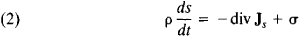Here, σ is the local entropy production per unit volume per unit time, and Js is the entropy flux, which is expressed in terms of the heat flux, diffusion flux, and the part of the stress tensor that is associated with nonequilibrium processes (that is, in terms of the viscous stress tensor Παβ).

In contrast to mass, energy, and momentum, entropy is not conserved but increases with time in a volume element at the rate σ as a result of irreversible processes. Furthermore, entropy can change through flow into or out of a volume element, a circumstance that is not connected with irreversible processes. In non-equilibrium thermodynamics the principle of entropy increase is expressed by the rate of entropy production being positive (σ > 0).

The rate of entropy production σ is determined solely by irreversible processes, such as diffusion, thermal conduction, and viscosity. In fact,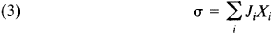where the Ji, are fluxes (for example, the diffusion flux Jk, heat flux Jq, or viscous stress tensor Παβ) and the Xi are the conjugate generalized forces, or affinities, that is, the gradients of the thermodynamic parameters that bring about deviation from the equilibrium state. To obtain a complete system of equations describing nonequilibrium processes, nonequilibrium thermodynamics expresses the fluxes of physical quantities in terms of the generalized forces by using phenomenological equations.

Phenomenological equations. Nonequilibrium thermodynamics proceeds on the assumption that where there are small deviations of a system from thermodynamic equilibrium the fluxes that arise are linearly dependent on the generalized forces and are described by phenomenological equations of the typewhere the Lik are called kinetic, phenomenological, or transport coefficients.

In “direct” processes the force Xk gives rise to the flux Jk. For example, a temperature gradient causes a heat flux (heat conduction), a concentration gradient produces a mass flux (diffusion), a velocity gradient causes a momentum flux (determines the viscosity), and an electric field gives rise to an electric current (electrical conduction). The kinetic coefficients characterizing these processes are proportional to the coefficients of thermal conductivity, diffusion, viscosity, and electrical conductivity, respectively; these coefficients are also referred to as kinetic, or transport, coefficients.

A force Xk may also give rise to a flux Ji, where ik. For example, a temperature gradient may bring about a mass flux in multicomponent systems; this phenomenon is known as thermal diffusion, or the Soret effect. In the Dufour effect, on the other hand, a concentration gradient causes a heat flux. Such processes represent coupling, or interference, effects and are characterized by the coefficient Lik, ik.

By making use of the phenomenological equations, we can obtain the following expression for the rate of entropy production:The Prigogine theorem states that, for fixed external conditions that impede the attainment of equilibrium, σ is at a minimum in stationary states. In the state of thermodynamic equilibrium, σ = 0. One of the basic theorems of nonequilibrium thermodynamics is the Onsager theorem, which asserts the symmetry of the kinetic coefficients in the absence of an external magnetic field and in the absence of rotation of the system as a whole: Lik = Lki.

Discontinuous systems. In the examples considered above, the thermodynamic parameters were continuous functions of the coordinates. Nonequilibrium systems are possible in which the thermodynamic parameters change discontinuously; such systems are said to be discontinuous, or heterogeneous. As an example, consider two gases enclosed in vessels connected by a capillary tube or membrane. If the temperatures T and chemical potentials μ of the gases in the vessels are not equal (T1 > T2 and μ1 > μ2), then the forces Xu and Xm, where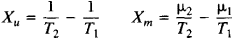produce between the two vessels the mass and energy fluxes Jm and Ju where Jm = L11Xm + L12Xu and Ju = L21Xm + L22XU. In this case nonequilibrium thermodynamics explains the occurrence of the thermomolecular pressure difference and the thermomechanical effect.

Since the fluxes and forces in the given example are scalar quantities, such processes may be said to be scalar. The fluxes and forces are vectors in the processes of diffusion, heat conduction, thermal diffusion, and the Dufour effect; accordingly, these processes may be referred to as vector processes. A viscous flow, with shear viscosity, may be called a tensor process, since the forces and fluxes are tensors. According to a theorem derived by P. Curie, in an isotropic medium the relations between forces and fluxes can be given by linear equations only if the forces and fluxes are of the same tensor dimensionality. In this case the phenomenological equations are greatly simplified.

Nonequilibrium thermodynamics provides a theoretical basis for the investigation of open systems and makes it possible to explain many nonequilibrium phenomena in conductors, such as thermoelectric effects, galvanomagnetic effects, and thermomagnetic effects. Nonequilibrium statistical thermodynamics, which bears the same relation to nonequilibrium thermodynamics as statistical thermodynamics does to thermodynamics, develops a statistical foundation for the laws of nonequilibrium thermodynamics and derives expressions for kinetic coefficients in terms of the parameters of the structure of matter.

### REFERENCES

Groot, S. R. de, and P. Mazur. Neravnovesnaia termodinamika. Moscow, 1964. (Translated from English.)
Prigogine, I. Vvedenie v termodinamiku neobratimykh protsessov. Moscow, 1960. (Translated from English.)
Denbigh, K. Termodinamika statsionarnykh neobratimykh protsessov. Moscow, 1954. (Translated from English.)
Haase, R. Termodinamika neobratimykh protsessov. Moscow, 1967. (Translated from German.)
Gyarmati, I. Neravnovesnaia termodinamika: Teoriia polia i variatsionnye printsipy. Moscow, 1974. (Translated from English.)

D. N. ZUBAREV

References in periodicals archive ?
2005) The nonequilibrium thermodynamics of small systems.
demonstrates that the application of the theory of internal variables in nonequilibrium thermodynamics results in hierarchical evolution equations.
They cover historical roots: from heat engines to cosmology, equilibrium thermodynamics, fluctuations and stability, linear nonequilibrium thermodynamics, and order through fluctuations.
This article focuses on some current theological issues, especially the misuse of the concept of entropy, and the current understanding of classical, statistical, and nonequilibrium thermodynamics.
1) For example, Gilbert states, "This move to the 'fluid and turbulent order' of nonequilibrium thermodynamics is a move to a system of bricolage where, as Serres puts it, 'nothing gets lost or wasted, but everything becomes useful'" (p.
The chaos characteristic of the nonlinear processes in nonequilibrium thermodynamics tends toward a state of order determined by what is known as an "attractor.
Although at first it appears that living systems violate the second law of thermodynamics in that they synthesize order from disorder, Schrodinger solved this problem by turning to nonequilibrium thermodynamics (NET).
Updates and new material are incorporated in chapters on fundamentals of equilibrium and nonequilibrium thermodynamics, transport and rate processes, using the Second Law, thermoeconomics, diffusion, heat and mass transfer, chemical reactions, coupled systems, membrane transport, biological systems, stability analysis, organized structures, and approaches, including a probabilistic approach.
balances) in nonequilibrium thermodynamics is a mathematical necessity in the case of higher order weakly nonlocal constitutive state spaces--overlooked by Gurtin in --and has a unifying power to understand the role of the Second Law in several seemingly different theories of continuum physics (see e.
The conference and also this special issue feature contributions in different fields of continuum physics such as Extended Irreversible Thermodynamics, Nonequilibrium Thermodynamics, Mesoscopic Continuum Physics, and Numerical Methods.
Therefore, their study is a challenge for nonequilibrium thermodynamics to better understand its basic concepts, its limits of application, and its frontiers.

Site: Follow: Share:
Open / Close